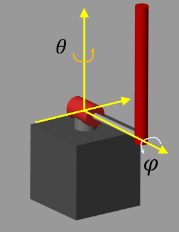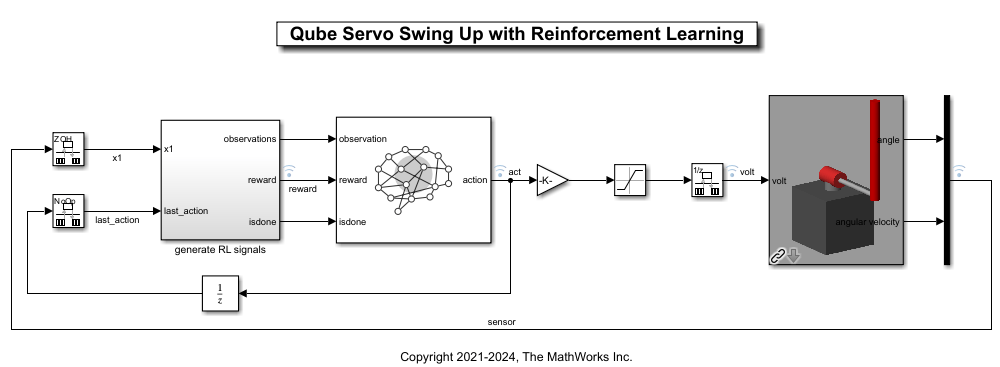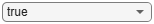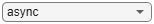# Train Reinforcement Learning Agents to Control Quanser QUBE Pendulum

This example trains reinforcement learning (RL) agents to swing up and control a Quanser QUBE™-Servo 2 inverted pendulum system.

### Inverted Pendulum Model

The Quanser QUBE-Servo 2 pendulum system is an implementation of a rotational inverted pendulum. It consists of a motor arm, which is actuated by a DC servo motor, with a freely swinging pendulum arm attached to its end. The system is challenging to control because it is underactuated, nonminimum phase, and highly nonlinear .

In this example, the pendulum system is modeled in Simulink® using Simscape™ Electrical™ and Simscape Multibody™ components. For this system:

• $\theta$ is the motor arm angle and $\phi$ is the pendulum angle.

• The motor arm angle is 0 radians when the arm is oriented horizontal and forward as shown in the diagram (counterclockwise is positive).

• The pendulum angle is 0 radians when the pendulum is oriented vertically downwards (counterclockwise is positive).

• Input of the plant model is a DC voltage signal for the motor. The voltage value ranges from –12 to 12 V.

• The pendulum and motor angles and angular velocities are measured by sensors.Load the parameters for this example using the `loadQubeParameters` helper script.

`loadQubeParameters`

```mdl = "rlQubeServo"; open_system(mdl)```### Control Structure

In this example, the RL agents generate reference trajectories, which are then passed to an inner control loop.

#### Outer-Loop Components

The outer loop of the control architecture injects the pendulum angle reference signal to the inner loop. It consists of the following reinforcement learning agents.

• Swing-up agent — A soft actor-critic (SAC) agent that computes reference angles for swinging up the pendulum arm.

• Mode-select agent — A proximal policy optimization (PPO) agent that performs a mode switching operation when the pendulum angle is close to the upright position ($\pi ±\pi /6$ radians).The mode-switching action (0 or 1) switches the outer-loop reference signal between the trajectory generated by the swing-up agent action and $\pi$ radians.

#### Inner-Loop Components

The inner-loop components compute the low-level control input ${\mathit{u}}_{\mathrm{motor}}$ (voltage) to stabilize the pendulum at the upright equilibrium point where the system is linearizable. Two proportional-derivative (PD) controllers form the inner-loop control system as shown in the following figure. For this example, the gains ${\mathit{P}}_{\theta },{\mathit{P}}_{\mathit{d}\theta },{\mathit{P}}_{\phi }$, and ${\mathit{P}}_{\mathit{d}\phi }$ were tuned to 0.1620, 0.0356, 40, and 2, respectively.### Train Swing-Up Agent

The swing-up agent in this example is modeled using the soft actor-critic (SAC) algorithm. For this agent:

• The environment is the pendulum system with the low-level controller. The mode-selection signal is always set to `1`.

• The observation is the vector $\left[\mathrm{sin}\theta ,\mathrm{cos}\theta ,\mathrm{sin}\phi ,\mathrm{cos}\phi ,\stackrel{˙}{\theta },\stackrel{˙}{\phi }\right]$.

• The action is the normalized outer-loop pendulum reference angle.

• The reward signal is as follows:

`$\begin{array}{l}\mathit{r}=-{\theta }^{2}-0.1{\left(\phi -\theta \right)}^{2}-0.1{\stackrel{˙}{\phi }}^{2}+\mathit{F}\\ \mathit{F}=\left\{\begin{array}{ll}100& \theta \in \pi ±\pi /6\text{\hspace{0.17em}\hspace{0.17em}}\mathrm{radians}\text{\hspace{0.17em}}\mathrm{and}\text{\hspace{0.17em}}\phi \in ±\pi \text{\hspace{0.17em}}\mathrm{radians}\\ 0& \mathrm{otherwise}\end{array}\end{array}$`

Open the Outer Loop Control block and set the Design mode parameter to `Swing up`. Doing so sets the mode-selection action to `1`, which configures the Switch block to pass the swing-up reference to the inner-loop controllers.

Alternatively, you can set this parameter using the following command.

`set_param(mdl + "/Outer Loop Control","DesignModeChoice","Swing up");`

Set the random seed for reproducibility.

`rng(0)`

Create the input and output specifications for the agent.

```swingObsInfo = rlNumericSpec([6,1]); swingActInfo = rlNumericSpec([1,1],LowerLimit=-1,UpperLimit=1);```

Create the environment interface.

```swingAgentBlk = ... mdl + "/Outer Loop Control/RL_Swing_Up/RL_agent_swing_up"; swingEnv = rlSimulinkEnv(mdl,swingAgentBlk,swingObsInfo,swingActInfo);```

The agent trains from an experience buffer of maximum capacity `1e6` by randomly selecting mini-batches of size `128`. The discount factor of `0.99` is close to `1` and therefore favors long term reward with respect to a smaller value. For a full list of SAC hyperparameters and their descriptions, see `rlSACAgent`. Specify the agent hyperparameters for training.

```swingAgentOpts = rlSACAgentOptions(... SampleTime=Ts,... TargetSmoothFactor=1e-3,... ExperienceBufferLength=1e6,... DiscountFactor=0.99,... MiniBatchSize=128);```

The actor and critic neural networks of the swing-up agent are updated by the Adam (adaptive moment estimation) optimizer with the following configuration. Specify the optimizer options.

```swingAgentOpts.ActorOptimizerOptions.Algorithm = "adam"; swingAgentOpts.ActorOptimizerOptions.LearnRate = 1e-4; swingAgentOpts.ActorOptimizerOptions.GradientThreshold = 1; for ct = 1:2 swingAgentOpts.CriticOptimizerOptions(ct).Algorithm = "adam"; swingAgentOpts.CriticOptimizerOptions(ct).LearnRate = 1e-3; swingAgentOpts.CriticOptimizerOptions(ct).GradientThreshold = 1; swingAgentOpts.CriticOptimizerOptions(ct).L2RegularizationFactor = 2e-4; end```

Create the agent.

```initOptions = rlAgentInitializationOptions(NumHiddenUnit=300); swingAgent = rlSACAgent(swingObsInfo,swingActInfo,initOptions,swingAgentOpts);```

To train the agent, first specify the training options using `rlTrainingOptions`. For this example, use the following options:

• Run each training for at most `1000` episodes, with each episode lasting at most `floor(Tf/Ts)` time steps.

• Stop training when the agent receives an average cumulative reward greater than `7500` over `50` consecutive episodes.

```swingTrainOpts = rlTrainingOptions(... MaxEpisodes=1000,... MaxStepsPerEpisode=floor(Tf/Ts),... ScoreAveragingWindowLength=50,... Plots="training-progress",... StopTrainingCriteria="AverageReward",... StopTrainingValue=7500);```

Train the agent using the `train` function. Training this agent is a computationally intensive process that takes several minutes to complete. To save time while running this example, load a pretrained agent by setting `doSwingTraining` to `false`. To train the agent yourself, set `doSwingTraining` to `true`.

```doSwingTraining = false; if doSwingTraining swingTrainResult = train(swingAgent,swingEnv,swingTrainOpts); else load("rlQubeServoAgents.mat","swingAgent"); end```### Train Mode-Select Agent

The mode-select agent in this example is modeled using the proximal policy optimization (PPO) algorithm. For this agent:

• The environment is the pendulum system with the low-level controller and the swing-up agent.

• The observation is the vector $\left[\mathrm{sin}\theta ,\text{\hspace{0.17em}}\mathrm{cos}\theta ,\text{\hspace{0.17em}}\mathrm{sin}\phi ,\text{\hspace{0.17em}}\mathrm{cos}\phi ,\text{\hspace{0.17em}}\stackrel{˙}{\theta },\text{\hspace{0.17em}}\stackrel{˙}{\phi }\right]$.

• The action is `0` or `1` which determines which reference signal is sent to the PD controller.

• The reward signal is as follows:

`$\begin{array}{l}\mathit{r}=-{\theta }^{2}+\mathit{G}\\ \mathit{G}=\left\{\begin{array}{ll}1& \theta \in \pi ±\pi /6\text{\hspace{0.17em}\hspace{0.17em}}\mathrm{radians}\\ 0& \mathrm{otherwise}\end{array}\end{array}$`

Open the Outer Loop Control block and set the Design mode parameter to `Mode select`.

Alternatively, you can set this parameter using the following command.

`set_param(mdl + "/Outer Loop Control","DesignModeChoice","Mode select");`

Create the input and output specifications for the agent.

```modeObsInfo = rlNumericSpec([6,1]); modeActInfo = rlFiniteSetSpec({0,1});```

Create the environment.

```modeAgentBlk = ... mdl + "/Outer Loop Control/RL_Mode_Select/RL_agent_select_mode"; modeEnv = rlSimulinkEnv(mdl,modeAgentBlk,modeObsInfo,modeActInfo);```

The mode-select agent trains by first collecting trajectories up to the experience horizon of `floor(Tf/Ts)` steps. It then learns from the trajectory data using a mini-batch size of `128`. The discount factor of `0.99` favors long-term reward and an entropy loss weight of `1e-4` facilities exploration during training.

Specify the hyperparameters for the agent. For more information on PPO agent options, see `rlPPOAgentOptions`.

```modeAgentOpts = rlPPOAgentOptions(... SampleTime=Ts,... DiscountFactor=0.99,... ExperienceHorizon=floor(Tf/Ts), ... MiniBatchSize=500, ... EntropyLossWeight=1e-4);```

The actor and critic neural networks of the mode-select agent are updated by the Adam optimizer with the following configuration. Specify the optimizer options.

```modeAgentOpts.ActorOptimizerOptions.LearnRate = 1e-4; modeAgentOpts.ActorOptimizerOptions.GradientThreshold = 1; modeAgentOpts.CriticOptimizerOptions.LearnRate = 1e-4;```

Create the agent.

```initOptions = rlAgentInitializationOptions(NumHiddenUnit=300); modeAgent = rlPPOAgent(modeObsInfo,modeActInfo,initOptions,modeAgentOpts);```

To train the agent, first specify the training options using `rlTrainingOptions`. For this example, use the following options:

• Run each training for at most `1000` episodes with each episode lasting at most `floor(Tf/Ts)` time steps.

• Stop training when the agent receives an average cumulative reward greater than `430` over `50` consecutive episodes.

```modeTrainOpts = rlTrainingOptions(... MaxEpisodes=10000,... MaxStepsPerEpisode=floor(Tf/Ts),... ScoreAveragingWindowLength=50,... Plots="training-progress",... StopTrainingCriteria="AverageReward",... StopTrainingValue=430);```

Train the agent using the `train` function. Training this agent is a computationally intensive process that takes several minutes to complete. To save time while running this example, load a pretrained agent by setting `doModeTraining` to `false`. To train the agent yourself, set `doModeTraining` to `true`.

```doModeTraining = false; if doModeTraining modeTrainResult = train(modeAgent,modeEnv,modeTrainOpts); else load("rlQubeServoAgents.mat","modeAgent"); end```### Simulation

Reset the random seed.

`rng(0)`

Configure the trained agents to use greedy policy during simulation.

```modeAgent.UseExplorationPolicy = false; swingAgent.UseExplorationPolicy = false;```

Ensure that the Outer Loop Control block is configured for mode-selection. Then, simulate the model.

```set_param(mdl + "/Outer Loop Control","DesignModeChoice","Mode select"); sim(mdl);```

View the performance of the agents in the Simulation Data Inspector. To open the Simulation Data Inspector, in the Simulink model window, on the Simulation tab, in the Review Results gallery, click Data Inspector.

In the plots:

• The measured values for $\theta$ (`sensor(1)`) and $\phi$ (`sensor(2)`) are stabilized at `0` and $\pi$ radians respectively. The pendulum is stabilized at the upright equilibrium position.

• The `action_RL_select_mode` signal shows the mode switching operation and the `action_RL_swing_up` signal shows the swing up reference angles.

• The low-level control input is shown by the `volt` signal.### References

 Cazzolato, Benjamin Seth, and Zebb Prime. ‘On the Dynamics of the Furuta Pendulum’. Journal of Control Science and Engineering 2011 (2011): 1–8.